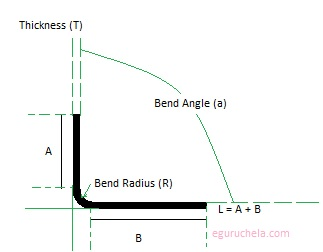### Bend Allowance Calculation

The calculator calculates the sheet metal bend allowance. It computes the bend allowance when forming sheet metal. The bend allowance means the amount of metal to be added to the total layout.
 Bend Angle (a) (degrees): Thickness (T) (inches): Inside Bend Radius (R) (Inches) : Bend Allowance(Change in length due to bending operation):

Bend allowance is a term which describes how much material is needed between two panels to accommodate a required bend. The bend allowances are important to calculate an exact length and then form it using the exact process to create the part. Once the part is formed, the part is measured and compared to the expected lengths and the bend allowance is adjusted as needed. The bend allowance is added to the length to determine the real cost of sheet metal items.
The Total length = Required length (A + B) + bend allowance as shown below in figure: